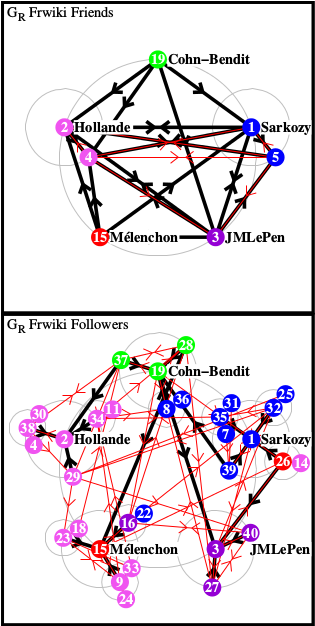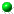Wikipedia mining of hidden links
between political leaders
by K.M.Frahm, K.Jaffres-Runser, and D.L.Shepelyansky
arXiv:1609.01948[cs.SI]Left: component G_qr of hidden links of reduced Google matrix G_R of 40 French politicians from French Wikipedia 2013 (politicians are ordered by PageRank); center: network of friends/followers (top/bottom) direction with up to 2 link levels (black, red arrows) in total G_R; right: same as in the center but for hidden links component G_qr (color coresponds to political parties)

 ` `Article download arXiv-v1 (Sept 2016): (here)All figs in tgz (here ) and zip (here ) formatsAll data for reduced Google matrix of politicians in tgz (here ) and zip (here ) formatsReadme about file structure (here)20 politicians of US from EN Wikipedia with matrix elements for: G_R; G_rr; G_pr; G_qr; G_qr friends order; G_qr followers order20 politicians of UK from EN Wikipedia with matrix elements for: G_R; G_rr; G_pr; G_qr; G_qr friends order; G_qr followers order40 politicians of DE from DE Wikipedia with matrix elements for: G_R; G_rr; G_pr; G_qr; G_qr friends order; G_qr followers order40 politicians of FR from FR Wikipedia with matrix elements for: G_R; G_rr; G_pr; G_qr; G_qr friends order; G_qr followers order20 politicians of RU from RU Wikipedia with matrix elements for: G_R; G_rr; G_pr; G_qr; G_qr friends order; G_qr followers order20 politicians of 2012 G20 Los Cabos summit from EN Wikipedia with matrix elements for: G_R; G_rr; G_pr; G_qr; G_qr friends order; G_qr followers order20 politicians of 2012 G20 Los Cabos summit from DE Wikipedia with matrix elements for: G_R; G_rr; G_pr; G_qr; G_qr friends order; G_qr followers order20 politicians of 2012 G20 Los Cabos summit from FR Wikipedia with matrix elements for: G_R; G_rr; G_pr; G_qr; G_qr friends order; G_qr followers order20 politicians of 2012 G20 Los Cabos summit from RU Wikipedia with matrix elements for: G_R; G_rr; G_pr; G_qr; G_qr friends order; G_qr followers orderData sets of Wikipedia networks EN, DE, FR, RU are available hereAll data for reduced Google matrix G*_R of politicians (CheiRank order) in tgz (here ) and zip (here ) formats This webpage is created at August 29, 2016 and is maintained by K.M.Frahm and D.L.Shepelyansky